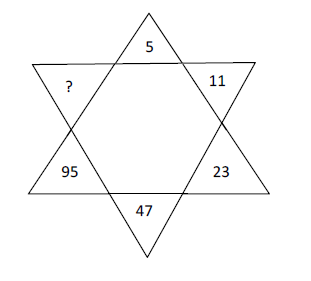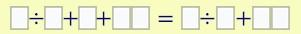# Easy Mathematical Puzzles - 2

Maths riddles and puzzles are based on various concepts of arithmetic, clocks, permutations, time and speed, etc. Solving these puzzles enhances your brain power as well as memory. Additionally, one of the most common puzzles are number puzzles. Here is a compilation of math brain teasers with answers and explanations. Each article consists of 10 simple maths puzzles.
Solve the given questions and check your mental abilities:
Q.1.Set A = (1,3,5,7------------297,299 -150 odd numbers). How many ways are there to choose exactly 18 numbers from set A such that their sum is 191?
Q.2.Vivek, a chain smoker, one day found it extremely difficult to go out to the market to get some cigarettes . He had some cigarette butts with him and he could pull tobacco out of some butts. 5 butts of tobacco make a cigarette. He collected the tobacco and made the cigarettes from the 121 butts available with him. How many cigarettes would he be able to smoke?
Q.3.Find the missing number:1. A. 189
2. B. 191
3. C. 3
4. D. 255
Q.4.In a faulty watch, the minute and the hour hand of a watch meet every 64 minutes. How much does the watch lose or gain time?
Q.5.The following set of boxes has to be filled in such a way that all the odd digits are utilized in the boxes on the left side while even digits are utilized on the right side boxes. The digits available are: 1, 2, 3, 4, 5, 6, 7, 8, and 9 to make the equation correct.Q.6.In an entrance exam, a student has to attempt 250 questions in 4 hours. Out of these 250 questions, 50 questions are on Quantitative Ability (QA). How many minutes should be spent on QA section if his mentor has suggested him that he must spend twice as much time on each maths problem as spent for every other question?
Q.7.Find the value of 4.4! + 5.5! + ......+n.n!
Q.8.The height of a certain flagpole is 33 feet. Grease is applied to the pole. A monkey attempts to climb the pole. It climbs 5 feet every second but slips down 4 ft in the next second. When will the monkey reach the top of the flagpole?
Q.9.Mangleshacharya was born in 1357 B.C. He had lived one-third of his life as a boy, one-fifth of his life as a youth, one-fourth of his life as a man and the remaining 39 years as an old man. Which year did he die?
Q.10.What is wrong with this proof?
2 = 1? ;
x = y
x2 = xy
x2 – y2 = xy – y2
(x + y) (x - y) = y (x - y)
x + y = y
2y = y
2 = 1